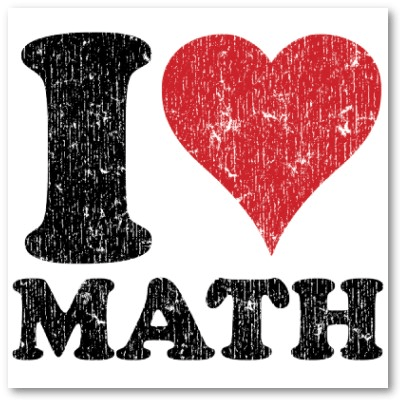# Simple Math: Graphical Multiplications

Date:  Jan 17, 2013

I came across this youtube today.  I found it very amusing.  And I think it is a good way to teach kids too.  And this is the way how Japanese teach their kids to do multiplications.  Very interesting way, I sua-gu, never learn this method before.  HaHaHa

http://lifehacker.com/5975917/quickly-multiply-big-numbers-the-japanese-way

So, I picked up a pen and pencil and trying to test it out.

Let’s give myself a not so simple one.  51×13=?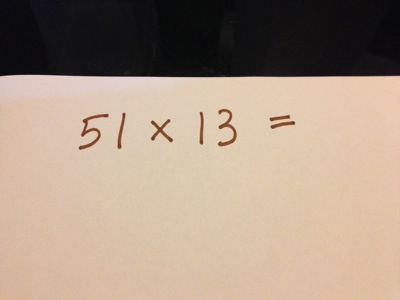Let’s draw 5 horizontal lines plus 1 horizontal line to represents “51”.  And leave some space between the 5 lines and the single line.Next draw the 1 single vertical line and 3 vertical lines intersecting the horizontal lines.  Again, leave wide space between the 1 line and 3 lines.  This is “13”Now, we start visualise and do the grouping.  Basically you can do three groupings.  This is the first group.  And then, you start counting the INTERSECTION points.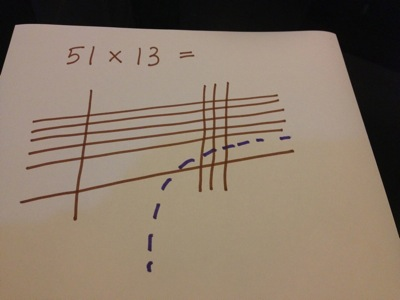1-2-3.  So, the single digit “3” is the addition of the intersections in the lower bottom right group.Then, you group the top left group.  And start counting the intersections points.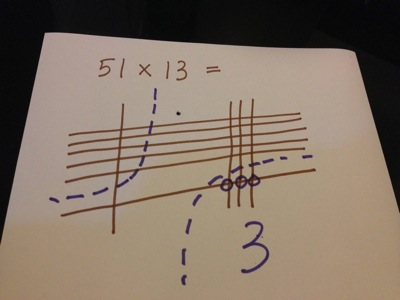1-2-3-4-5.  And you have a “5” in the top left group.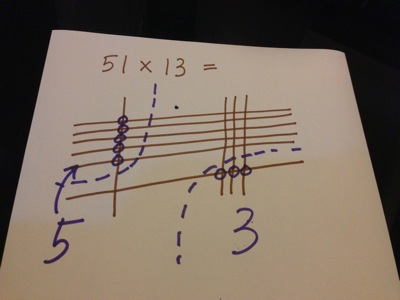Then, there is only one diagonal group.  Which is what I have circle in the diagram, so, you count the intersection points.  There are 1 and there are 15 so, add up them to become “16”.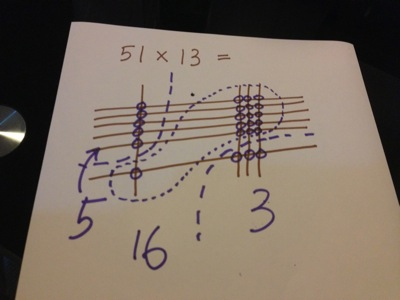Every group must be a “Single Digit” number.  So, you cannot have “16” in the middle.  So, what you do, you move the “ten” or “1” into the left or top group as a single digit and add them up.  进位.  So, 5 + 1 (move from the middle group) is equal 6.So, the answer is 663.  WOW.Let’s check it again.  Yup, no doubt.  51 x 13 = 663So, if you do a simple single digit multiplication, it works too.  Just count the intersections.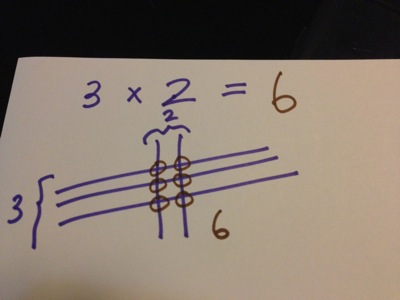So, if you do a double digit Vs single digit multiplication, it works as well.  There is no diagonal group, only two groups, top and bottom.  15 x 3 = 45.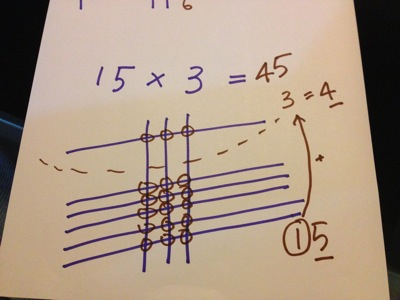Math is a strange thing.  You love and you hate it.  But the world cannot live without it.